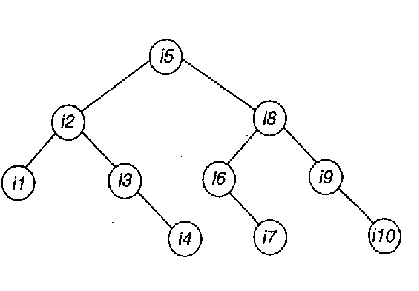# Data Structures - Sorting & Searching

36:

The average number of comparisons performed by the merge sort algorithm, in merging two sorted liss of length 2 is

 A. 8/3 B. 8/5 C. 11/7 D. 11/6 Answer Report Discuss Option: A Explanation : Merge-sort combines two given sorted lists into one sorted list. For this problem let the final sorted order be- 1, b, c, d. The two lists (of length two each) should fall into one of the following 3 categories. (i) a, b and c,d  (ii) a, c and b, d  (iii) a, d and b, c The number of comparisons needed in each case will be 2, 3, 3. So, average number of comparisons will be (2=3+3)/3= 8/3 Here is a better way of doing; Let list L1 have the items a,c and L2 have the items b,d.The tree drawn below, depicts the different possible cases. (a & b means a is compared with b. If a is smaller, the edge will be labeled a. The number within a circle, beside the leaf nodes, is the number of comparisons, needed to reach it.) From the tree, we find there are 6 possible ways, total number of comparisons needed is 3+3+2+2+3+3=16. So, average number of comparisons is 16/6= 8/3.   Click on Discuss to view users comments. Write your comments here:
37:

Which of the following sorting methods will be the best if number of swappings done, is the only measure of efficienty?

 A. Bubble sort B. Selection sort C. Insertion sort D. Quick sort Answer Report Discuss Option: B Explanation : Because in selection sort algorithm we randomly access data rather than a list in which we can easily swap data which we want to swap and it takes less time. So, answer is 'B' Click on Discuss to view users comments. Write your comments here:
38:

You are asked to sort 15 randomly generated numbers. You should prefer

 A. bubble sort B. Selection sort C. Insertion sort D. Quick sort Answer Report Discuss Option: A Explanation : Click on Discuss to view users comments. Write your comments here:
39:

As part of the maintenance work, you are entrusted with the work of rearranging the library books in a shelf in proper order, at the end of each day. The ideal choice will be

 A. bubble sort B. insertion sort C. selection sort D. heap sort Answer Report Discuss Option: B Explanation : Click on Discuss to view users comments. rama said: (9:02pm on Wednesday 13th February 2013) why only insertion sort? Debananda Mahata said: (1:26am on Sunday 17th December 2017) I think insertion Sort (B) will be right answer.looking at the question in its sense that you have to arrange books in a shelf you just need to pick the book and find its place using insertion sort. Keep repeating till end. Write your comments here:
40:

The maximum number of comparisons needed to sort 7 items using radix sort is (assume each item is 4 digit decimal number)

 A. 280 B. 40 C. 47 D. 38 Answer Report Discuss Option: A Explanation : The maximum number of comparison is number of items ´ radix ´ number of digits i.e., 7´10´4 = 280. Click on Discuss to view users comments. Write your comments here: